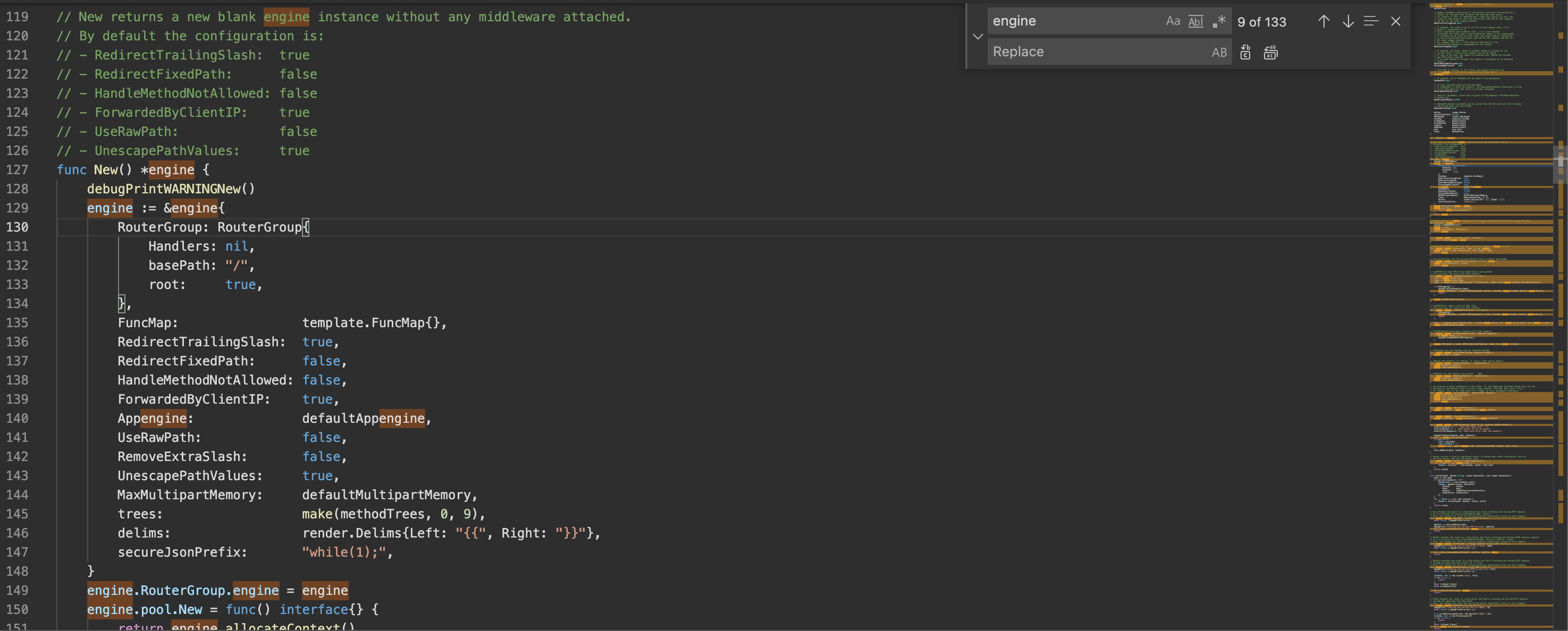# String Matching: Naive Algorithm

Some programs that you use everyday need to find occurrences of a string in a text.
Think about your preferred IDE or simply any text-editing program.
When you misspelling a variable name and you want to fix this, you need to find all the part of the text where the name is present and substitute it.When you type ctrl + F in vscode you are going to do a string matching

String Matching algorithms are widely used in the field of Bioinformatics for detect patterns in DNA or RNA sequences since they are represented digitally as strings over a well-defined alphabet.

The string matching (or pattern matching) problem is define as follows.

We assume that the text T is an array of length n and the pattern P is an array of m elements.
We say that pattern P occurs with shifts s in text T if

• 0 ≤ s ≤ n-m, and
• T[s+1 : s+m] = P[1 : m]

A shift is valid only if pattern P occurs with shift s in text T.
We can say that the string matching problem is the problem of finding all valid shifts with which the pattern P occurs in a text T.

# The Naive Algorithm

Let’s see now a very simple (and inefficient) algorithm for string matching, the so called naive string-matching algorithm.

We use a for loop from 0 to n-m (respectively, the length of the text T and the length of the pattern P) to checks if P is equal to the substring of S taken from s+1 and s+m.

# Pseudocode

`NaiveStringMatcher(T,P):   n = length(T)   m = length(P)   FOR s = 0 to n-m do:      IF P[1 : m] == T[s+1 : s+m]:         print "Pattern occurs with shift " s`

The pattern slides over the text and if the pattern is found in the text starting from s (the if statement is true), we print out the shift s.

This is an inefficient algorithm for long text. Why?
Because for every possible n-m+1 shift we have to compare all the character of P with the m character of T[s+1 : s+m].
The worst case running time is Θ((n-m+1)*m).
If m is equal to n/2, the running time is Θ(n²).

# Golang implementation

Now we see how this naive algorithm can be implemented in golang.

`func NaiveStringMatching(text string, pattern string) {   n := len(text)   m := len(pattern)   for s := 0; s < (n-m)+1; s++ {      if pattern == text[s:s+m] {         fmt.Println("Pattern occurs with shift", s)      }   }}`

EXAMPLE OF EXECUTION

With Text

And Pattern “selva”, the output of the above algorithm is:

`Pattern occurs with shift 56Pattern occurs with shift 148Pattern occurs with shift 154`

This means that we can found the string “selva” in the text T three time:

• From position 56 to 56+m (where m in this case is 5), T[56 : 56 + 5]
• From position 148 to 148+5, T[148 : 148 + 5]
• From 154 to 154 + 5, T[154 : 154 + 5]
`func main() {   text := "Nel mezzo del cammin di nostra vita mi ritrovai per una      selva oscura, ché la diritta via era smarrita. Ahi quanto a dir qual era è cosa dura esta selva selvaggia e aspra e forte che nel pensier rinova la paura!"   pattern := "selva"   NaiveStringMatching(text, pattern)   fmt.Println("T[56:56+5] -> ", text[56:56+5])   fmt.Println("T[146:148+5] -> ", text[148:148+5])   fmt.Println("T[154:154+5] -> ", text[154:154+5])}// OUTPUT: go run main.goPattern occurs with shift 56Pattern occurs with shift 148Pattern occurs with shift 154T[56:56+5] ->  selvaT[146:148+5] ->  selvaT[154:154+5] ->  selva`

# And now?

There are other types of string matching algorithm that works better.
The main defect of the Naive Algorithm is that it ignores the information gained on a value of s when it consider other values of s.
In the next posts, we will see other algorithms, like the Knuth-Morris-Pratt algorithm and another one that uses finite automata.